# Class 10 Science Chapter 10 Light Reflection and Refraction

NCERT Solutions For Class 10 Science Chapter 10 Light Reflection and Refraction. Question Answer.

Question 1.

Define principal focus of a concave mirror.
It is a point on the principal axis at which parallel rays of light travelling close to principal axis actually converge after reflection.

Question 2.
The radius of curvature of a spherical mirror is 20 cm. What is its focal length?
F o c a l l e n g t h = R a d i u s o f c u r v a t u r e 2 = 20 2 = 10 c m

Question 3.
Name a mirror that can given an erect and enlarged image of an object.
Concave mirror

Question 4.
Why do we prefer a convex mirror as a rear-view mirror in vehicles?  [2011, 2012]
This is because a convex mirror forms diminished and erect images. It also provides a wider view of the vehicle.

Question 5.
Find the focal length of a concave mirror whose radius of curvature is 32 cm.
F o c a l l e n g t h = R a d i u s o f c u r v a t u r e 2 = 32 2 = 16 c m

Question 6.
A concave mirror produces three times magnified [enlarged] real image of an object placed at 10 cm in front of it. Where is the image located?
Given: u = -10 cm, m = -3 and v = ?Question 7.
A ray of light travelling in air enters obliquely into water. Does the light ray bend towards the normal or away from the normal? Why?
The light ray bends towards the normal when it enters into water. Because water is an optically denser medium.

Question 8.
Light enters from air to glass having refractive index 1.5. What is the speed of light in the glass? The speed of light in vacuum is 3 x 10 8 m s -1
Given: refractive index, μ = 1.5, speed of light in vacuum
c = 3 x 10 8 m s -1 v = ?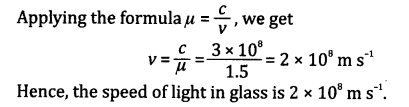Question 9.
Find out from Table 10.3 [NCERT] the medium having highest optical density. Also, find the medium with lowest optical density.
From Table 10.3, diamond has highest refractive index (n = 2.42). So it has highest optical density.
Air has lowest refractive index [n = 1.0003]. So, it has lowest optical density.

Question 10.
You are given kerosene, turpentine and water. In which of these does the light travel fastest? Use the information given in Table 10.3 [NCERT],
The refractive index for:
kerosene = 1.44, turpentine = 1.47 and water = 1.33
Here, the refractive index of water is lowest, so this is an optically rarer medium than kerosene and turpentine. So light travels fastest in water.

Question 11.
The refractive index of diamond is 2.42. What is the meaning of this statement?  [2012,2013]
This means that the speed of light in air and that in diamond is equal to 2.42.

Question 12.
Define 1 dioptre power of a lens.  
1 dioptre is the power of a lens of focal length one metre.

Question 13.
A convex lens forms a real and inverted image of a needle at a distance of 50 cm from it. Where is the needle placed in front of the convex lens if the image is equal to the size of the object. Also, find the power of the lens.
Given: v = 50 cm, u = ?, P = ?
Since the image is equal to the size of the object, so the object is placed at a distance twice the focal length of the lens, i.e.
-u = v = 2f= 50 cm
∴ u = -50 cm and f= 25 cm
Hence, the needle is placed at a distance of 50 cm in front of the lens.
Nowpower,  P = 100 f ( c m ) = 100 25 = + 4 D

Question 14.
Find the power of a concave lens of focal length 2 m.
Given: Focal length, f = -2 m = -200 cm
∴ Power,  P = 100 f = 100 200 = 0.5 D

Chapter End Questions

Question 1.
Which one of the following materials cannot be used to make a lens?
(a) Water
(b) Glass
(c) Plastic
(d) Clay
(d) Clay

Question 2.
The image formed by a concave mirror is observed to be virtual, erect and larger than the object. Where should be the position of the object?
(a) Between the principal focus and the centre of curvature
(b) At the centre of curvature
(c) Beyond the centre of curvature
(d) Between the pole of the mirror and its principal focus
(d) etween the pole of the mirror and its principal focus

Question 3.
Where should an object be placed in front of a convex lens to get a real image of the size of the object?
(a) At the principal focus of the lens
(b) At twice the focal length
(c) At infinity
(d) Between the optical centre of the lens- and its principal focus
(b) At twice the focal length

Question 4.
A spherical mirror and a thin spherical lens have each a focal length of -15 cm. The mirror and the lens are likely to be
(a) both concave
(b) both convex
(c) the mirror is concave and the lens is convex
(d) the mirror is convex and the lens is concave
(a) both concave

Question 5.
No matter how far you stand from a mirror, your image appears erect. The mirror is likely to be
(a) plane
(b) concave
(c) convex
(d) either plane or convex
(d) either plane or convex

Question 6.
Which of the following lenses would you prefer to use while reading small letters found in a dictionary?
(a) A convex lens of focal length 50 cm
(b) A concave lens of focal length 50 cm
(c) A convex lens of focal length 5 cm
(d) A concave lens of focal length 5 cm
(c) A convex lens of focal length 5 cm

Question 7.
We wish to obtain an erect image of an object, using a concave mirror of focal length 15 cm. What should be the range of distance of the object from the mirror? What is the nature of the image? Is the image larger or smaller than the object? Draw a ray diagram to show the image formation in this case.
The range of distance of the object from the mirror should be between focus and pole.
Image is virtual, erect and larger than the object. The ray diagram showing the image formation
is given below.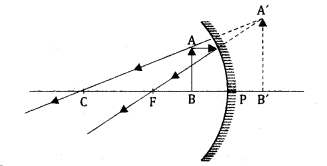Question 8.
Name the type of mirror used in the following situations:  [2012,2013]
(b) Rear-view of a vehicle
(c) Solar furnace
(a)  Concave mirror, as it can give an inverted and enlarged image which meet at infinity,
(b)  Convex mirror, as it can give an erect and diminished image within the focal length.
(c)  Concave mirror, as it can converge all the parallel beam of light rays at one point.

Question 9.
One-half of a convex lens is covered with a black paper. Will this lens produce a complete image of the object? Verify your answer experimentally. Explain your observation.  
Yes, it will produce a complete image of the object. This can be verified experimentally as follows:
Procedure:

1. Take a metre scale and place it horizontally against a white or light coloured wall with 0 cm end touching the wall.
2. Take a convex lens and focus the light from a distant object, say a tree, by moving the lens to and fro along the metre scale.
3. A sharp image of the tree is formed on the wall at a distance equal to the focal length of the lens.
4. Cover lower or upper half of the lens with black paper.
5. Again focus the light from the same tree on the wall.
A less bright image of the tree is formed on the wall from the same point on the metre scale.Observation: It is observed that when half of the lens is covered, its focal length remains the same. Only the intensity of light entering the lens decreases due to which the brightness of the image also decreases.
Diagrammatically, it can be represented as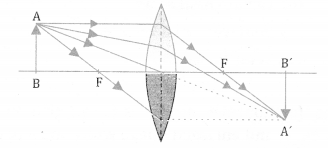Question 10.
An object 5 cm in length is held 25 cm away from a converging lens of focal length 10 cm. Draw the ray diagram and find the position, size and the nature of the image formed.  
The ray diagram is shown below: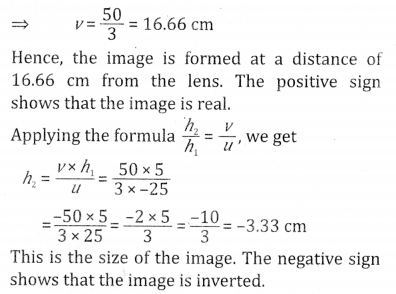Question 11.
A concave lens of focal length 15 cm forms an image 10 cm from the lens. How far is the object placed from the lens. Draw the ray diagram.
The complete ray diagram is shown below: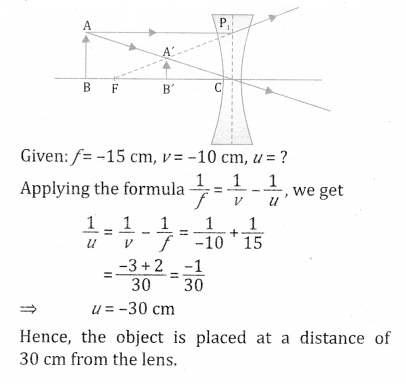Question 12.
An object is placed at a distance of 10 cm from a convex mirror of focal length 15 cm. Find the position and nature of the image.  
Given: u = -10 cm, f = 15 cm and v = ?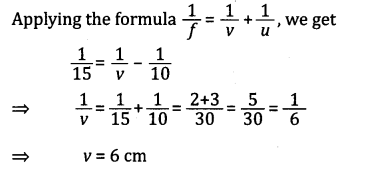Hence, the image is formed behind the mirror. It is erect, virtual and smaller than the object.

Question 13.
The magnification produced by a plane mirror is +1. What does this mean?   [2011,2014]
∵  m = h 2 h 1 = + 1
∴ h 2 = h 1
Therefore, the size of image is equal to the size of object and positive sign shows that the image is erect.

Question 14.
An object 5.0 cm in length is placed at a distance of 20 cm in front of a convex mirror of radius of curvature 30 cm. Find the position of the image, its nature and size.
Given: h 1 = 5.0 cm, u = -20 cm, R = 30 cmQuestion 15.
An object of size 7.0 cm is placed at a distance of 27 cm in front of a concave mirror of focal length 18 cm. At what distance from the mirror should a screen be placed, so that a sharp focussed image can be obtained? Find the size and nature of the image.
Given: h 1 = 7 cm, u = -27 cm, f = -18 cm,
v = ?Question 16.
Find the focal length of a lens of power -2.0 D. What type of lens is this?